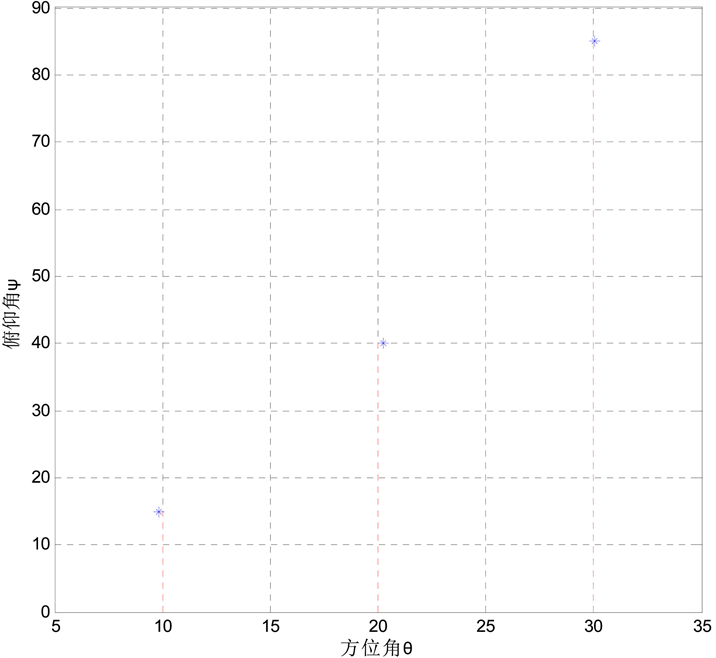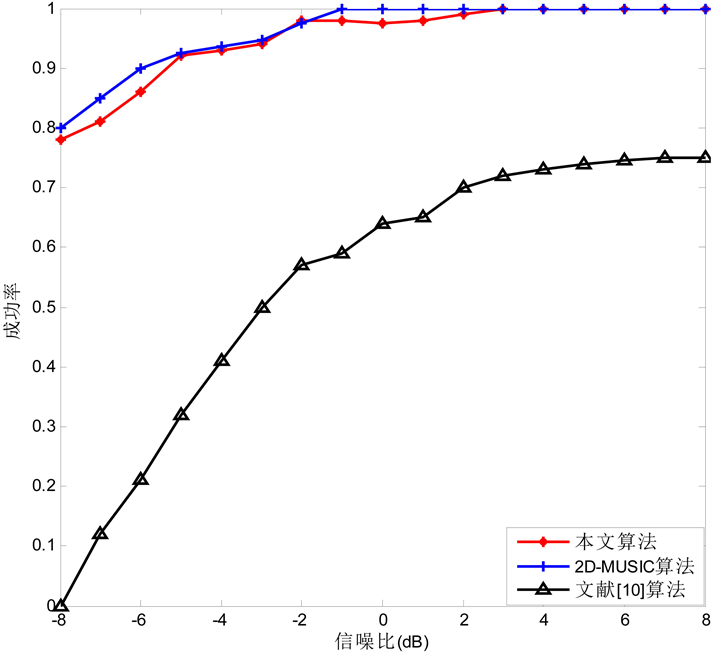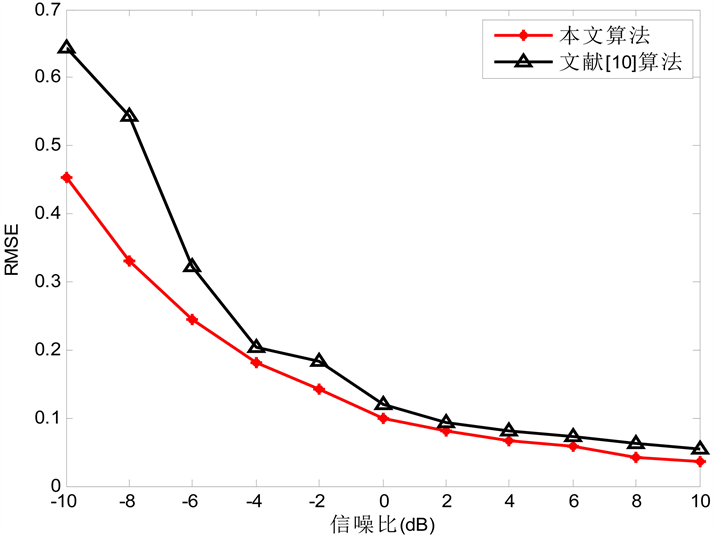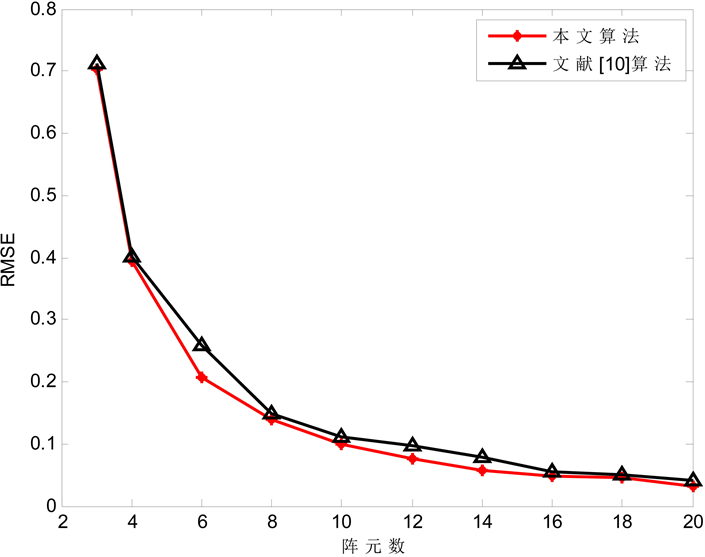﻿ 大规模MIMO系统中基于模等式约束的降维去相干DOA估计

# 大规模MIMO系统中基于模等式约束的降维去相干DOA估计Modulus Equation Constraints Based Decoherent Dimension Reduction 2-D DOA Estimation for Massive MIMO Systems

Abstract: There are large numbers of coherent signals in massive MIMO system. Simple decoherence pro-cessing leads to the lower accuracy of the 2-D DOA (Two-Dimensional Direction of Arrival). This paper will propose a modulus equation constraints based dimension reduction MUSIC algorithm which can greatly improve the performance of two-dimensional DOA estimation of coherent signals. The algorithm transforms the two-dimensional DOA estimation problem into an optimization problem, and uses the modulus equation constraints to define the additional conditions and impose strong constraints on the direction vector so that the optimization equation is solved more close to the optimal solution. The results of theoretical analysis and simulation experiments show that the proposed DOA algorithm has high reliability and precision. Such algorithm is able to meet the requirements of DOA estimation performance in massive MIMO system, and can also provide high feasibility and practicability.

1. 引言

2. 信号模型与相关性分析

$r=\frac{\mathrm{cov}\left({s}_{1},{s}_{2}\right)}{\sqrt{D\left({s}_{1}\right)D\left({s}_{2}\right)}}=\frac{E\left\{\left[{s}_{1}-E\left\{{s}_{1}\right\}\right]\left[{s}_{2}-E\left\{{s}_{2}\right\}\right]\right\}}{\sqrt{E\left\{{\left[{s}_{1}-E\left\{{s}_{1}\right\}\right]}^{2}\right\}E\left\{{\left[{s}_{2}-E\left\{{s}_{2}\right\}\right]}^{2}\right\}}}$ (1)

$r=0$ 时，信号不相关；当 $0 时，信号相关；当 $r=1$ 时，信号相干。

$X\left(t\right)={A}_{x}S\left(t\right)+{N}_{x}\left(t\right)$ (2)

$Y\left(t\right)={A}_{y}S\left(t\right)+{N}_{y}\left(t\right)$ (3)Figure 1. Schematic diagram of l-shaped array antenna

$\begin{array}{l}{a}_{x}\left({\theta }_{k}\right)={\left[{a}_{x,1}\left({\theta }_{k}\right),{a}_{x,2}\left({\theta }_{k}\right),\cdots ,{a}_{x,M}\left({\theta }_{k}\right)\right]}^{\text{T}}={\left[1,{\text{e}}^{-j2\text{π}d\mathrm{cos}{\phi }_{1}\mathrm{sin}{\theta }_{1}/\lambda },\cdots ,{\text{e}}^{-j2\text{π}\left(M-1\right)d\mathrm{cos}{\phi }_{k}\mathrm{sin}{\theta }_{k}/\lambda }\right]}^{\text{T}}\\ {a}_{y}\left({\phi }_{k}\right)={\left[{a}_{y,1}\left({\phi }_{k}\right),{a}_{y,2}\left({\phi }_{k}\right),\dots {a}_{y,M}\left({\phi }_{k}\right)\right]}^{\text{T}}={\left[1,{\text{e}}^{-j2\text{π}d\mathrm{sin}{\phi }_{1}\mathrm{sin}{\theta }_{1}/\lambda },\cdots ,{\text{e}}^{-j2\text{π}\left(M-1\right)d\mathrm{sin}{\phi }_{k}\mathrm{sin}{\theta }_{k}/\lambda }\right]}^{\text{T}}\end{array}$ (4)

$\left\{\begin{array}{l}\mathrm{cos}{\alpha }_{k}=\mathrm{cos}{\phi }_{k}\mathrm{sin}{\theta }_{k}\\ \mathrm{cos}{\beta }_{k}=\mathrm{sin}{\phi }_{k}\mathrm{sin}{\theta }_{k}\end{array}$ (5)

3. 去相干算法

${x}_{k}\left(t\right)=\left[{x}_{k}\text{\hspace{0.17em}}\text{\hspace{0.17em}}{x}_{k+1}\text{\hspace{0.17em}}\text{\hspace{0.17em}}\cdots \text{\hspace{0.17em}}\text{\hspace{0.17em}}{x}_{k+m-1}\right]={A}_{xk}{D}^{\left(k-1\right)}s\left(t\right)+{n}_{k}\left(t\right)$ (6)

${R}_{xk}={A}_{xk}{\eta }^{\left(k-1\right)}{R}_{s}{\left({D}^{\left(k-1\right)}\right)}^{H}{A}_{xk}^{H}+{\sigma }^{2}I$ (7)

${R}_{x}^{f}=\frac{1}{p}{\sum }_{i=1}^{p}{R}_{ip}={A}_{xk}{R}_{xs}^{f}{A}_{xk}^{H}+{\sigma }^{2}I$ (8)

${R}_{x}^{b}=\frac{1}{p}{\sum }_{i=1}^{p}{R}_{xp}^{b}={A}_{xk}{R}_{xs}^{b}{A}_{xk}^{H}+{\sigma }^{2}I$ (9)

${R}_{x}^{fb}=\frac{1}{2}\left({R}_{x}^{f}+{R}_{x}^{b}\right)$ (10)

${R}_{y}^{fb}=\frac{1}{2}\left({R}_{y}^{f}+{R}_{y}^{b}\right)$ (11)

$Z={\left[{R}_{x}^{fb}\text{\hspace{0.17em}}\text{\hspace{0.17em}}{R}_{y}^{fb}\right]}^{\text{T}}$ (12)

4. 降维MUSIC算法

${P}_{2dmusic}=\frac{1}{{\left[{a}_{x}\left({\theta }_{k}\right)\otimes {a}_{y}\left({\phi }_{k}\right)\right]}^{H}{U}_{n}{U}_{n}^{H}\left[{a}_{x}\left({\theta }_{k}\right)\otimes {a}_{y}\left({\phi }_{k}\right)\right]}$ (13)

$Q\left({\theta }_{k},{\phi }_{k}\right)={\left[{a}_{x}\left({\theta }_{k}\right)\otimes {a}_{y}\left({\phi }_{k}\right)\right]}^{H}{U}_{n}{U}_{n}^{H}\left[{a}_{x}\left({\theta }_{k}\right)\otimes {a}_{y}\left({\phi }_{k}\right)\right]$ (14)

$Q\left({\theta }_{k},{\phi }_{k}\right)={a}_{y}^{H}\left({\phi }_{k}\right){\left[{a}_{x}\left({\theta }_{k}\right)\otimes {I}_{M}\right]}^{H}{U}_{n}{U}_{n}^{H}\left[{a}_{x}\left({\theta }_{k}\right)\otimes {I}_{M}\right]{a}_{y}\left({\phi }_{k}\right)$ (15)

$G\left({\theta }_{k}\right)={\left[{a}_{x}\left({\theta }_{k}\right)\otimes {I}_{M}\right]}^{H}{U}_{n}{U}_{n}^{H}\left[{a}_{x}\left({\theta }_{k}\right)\otimes {I}_{M}\right]$ (16)

$\mathrm{min}{a}_{y}^{H}\left({\phi }_{k}\right)G\left({\theta }_{k}\right){a}_{y}\left({\phi }_{k}\right)=\mathrm{min}Q\left({\theta }_{k},{\phi }_{k}\right)$ (17)

${a}_{y}\left(y\right)=\mathrm{exp}\left[-j2\text{π}d\left(m-1\right)\mathrm{cos}{\beta }_{k}/\lambda \right]={\left[\mathrm{exp}\left(-j2\text{π}d\mathrm{cos}{\beta }_{k}/\lambda \right)\right]}^{m-1}={a}_{y}^{y-1}=\left\{\begin{array}{l}1,\text{\hspace{0.17em}}\text{\hspace{0.17em}}\text{\hspace{0.17em}}\text{\hspace{0.17em}}\text{\hspace{0.17em}}\text{\hspace{0.17em}}\text{\hspace{0.17em}}\text{\hspace{0.17em}}\text{\hspace{0.17em}}\text{\hspace{0.17em}}\text{\hspace{0.17em}}\text{\hspace{0.17em}}\text{\hspace{0.17em}}\text{\hspace{0.17em}}\text{\hspace{0.17em}}\text{\hspace{0.17em}}\text{\hspace{0.17em}}\text{\hspace{0.17em}}\text{\hspace{0.17em}}\text{\hspace{0.17em}}\text{\hspace{0.17em}}\text{\hspace{0.17em}}\text{\hspace{0.17em}}\text{\hspace{0.17em}}m=1\\ {a}_{y}\left(2\right),\text{\hspace{0.17em}}\text{\hspace{0.17em}}\text{\hspace{0.17em}}\text{\hspace{0.17em}}\text{\hspace{0.17em}}\text{\hspace{0.17em}}\text{\hspace{0.17em}}\text{\hspace{0.17em}}\text{\hspace{0.17em}}\text{\hspace{0.17em}}\text{\hspace{0.17em}}\text{\hspace{0.17em}}\text{\hspace{0.17em}}\text{\hspace{0.17em}}\text{\hspace{0.17em}}\text{ }\text{\hspace{0.17em}}m=2\\ {a}_{y}\left(2\right){a}_{y}\left(y-1\right),\text{\hspace{0.17em}}\text{\hspace{0.17em}}\text{\hspace{0.17em}}m\ge 3\end{array}$ (18)

5. 改进的降维MUSIC (MRD-MUSIC)算法

$\left\{\begin{array}{l}{\mathrm{min}}_{w}{a}_{y}^{H}G\left({\theta }_{k}\right){a}_{y}\\ \text{s}\text{.t}\text{.}\text{\hspace{0.17em}}{‖{a}_{y}\left({\phi }_{k}\right)‖}^{2}=M\end{array}$ (19)

$L\left({a}_{y},{\theta }_{k}\right)={a}_{y}^{H}G\left({\theta }_{k}\right){a}_{y}-\lambda \left({a}_{y}^{H}{a}_{y}-M\right)$ (20)

$\frac{\partial }{\partial {a}_{y}}L\left({a}_{y},{\theta }_{k}\right)=2G\left({\theta }_{k}\right)\cdot {a}_{y}-2\lambda \cdot {a}_{y}=0$ (21)

$G\left({\theta }_{k}\right)\cdot {a}_{y}=\lambda \cdot {a}_{y}$ (22)

${a}_{y}^{H}\cdot G\left({\theta }_{k}\right)\cdot {a}_{y}=\lambda \cdot {a}_{y}^{H}\cdot {a}_{y}=\lambda \cdot M$ (23)

${a}_{y}={P}_{\mathrm{min}}\left[G\left({\theta }_{k}\right)\right]$ (24)

${\stackrel{^}{\theta }}_{k}=\mathrm{arg}\mathrm{min}{P}_{\mathrm{min}}^{H}\left[G\left({\theta }_{k}\right)\right]\cdot G\left({\theta }_{k}\right){P}_{\mathrm{min}}\cdot \left[G\left({\theta }_{k}\right)\right]=\mathrm{arg}\mathrm{max}\frac{1}{{P}_{\mathrm{min}}^{H}\left[G\left({\theta }_{k}\right)\right]\cdot G\left({\theta }_{k}\right){P}_{\mathrm{min}}\cdot \left[G\left({\theta }_{k}\right)\right]}$ (25)

6. 实验仿真与结果分析

$\text{RMSE}=\frac{1}{K}{\sum }_{k=1}^{K}\sqrt{\frac{1}{Num}{\sum }_{num=1}^{Num}{\left({\stackrel{^}{\theta }}_{k,num}-{\theta }_{k}\right)}^{2}+{\left({\stackrel{^}{\phi }}_{k,num}-{\phi }_{k}\right)}^{2}}$ (26)

DOA精度：令信噪比SNR范围从−10 dB到10 dB，快拍数为1000，进行500次蒙特卡罗仿真实验。将文献  去相干算法和本文算法的均方根误差曲线进行对比。对比如图5所示。Figure 3. Estimation of pitch angle and azimuth angleFigure 4. Comparison of success rateFigure 5. The change curve of RMSE with SNRFigure 6. The variation curve of RMSE with array elements

7. 结论

NOTES

*通讯作者。

 Xu, K., Nie, W., Feng, D., et al. (2016) A Multi-Direction Virtual Array Transformation Algorithm for 2D DOA Estimation. Signal Processing, 125, 122-133.

 Tayem, N. and Kwon, H.M. (2005) L-Shape 2-Dimensional Arrival Angle Estimation with Propagator Method. IEEE Transactions on Antennas and Propagation, 53, 1622-1630.
https://doi.org/10.1109/TAP.2005.846804

 王伟, 王小萌, 等. 基于MUSIC算法的L型阵列MIMO雷达降维DOA估计[J]. 电子与信息学报, 2014, 36(8): 1954-1959.

 Liang, J.L. and Liu, D. (2010) Joint Elevation and Azimuth Direction Finding Using L-Shaped Array. IEEE Transations on Antennas and Propagation, 58, 2136-2141.
https://doi.org/10.1109/TAP.2010.2046838

 Cuangmin, W., Jingmin, X. Nanning, Z., et al. (2011) Computationally Efficient Subspace-Based Method for Two-Dimensional Direction Estimation with L-Shaped Array. IEEE Transactions on Signal Process, 59, 3197-3212.
https://doi.org/10.1109/TSP.2011.2144591

 Nie, X. and Li, L.P. (2014) A Computationally Efficient Subspace Algorithm for 2-D DOA Estimation with L-Shaped Array. IEEE Transactions on Signal Processing Letters, 21, 971-974.
https://doi.org/10.1109/LSP.2014.2321791

 Wei, Y.S. and Guo, X.J. (2014) Pair-Matching Method by Signal Covariance Matrices for 2D-DOA Estimation. IEEE Antennas Wireless Propagaton Letters, 13, 1199-1202.

 杨艳飞, 高健, 张兴敢, 等. 一种基于L型阵列的改进的二维DOA估计方法[J]. 南京大学学报, 2016, 52(5): 953-959.

 梁浩, 崔琛, 代林, 等. 基于ESPRIT算法的L型阵列MIMO雷达降维DOA估计[J]. 电子与信息学报, 2015, 37(8): 1823-1835.

 张艳萍, 赵玉垒, 孙心宇. 一种L型阵列的相干分布降维DOA估计方法[J]. 计算机应用研究, 2016, 33(5): 1477-1480.

 熊波, 李国林, 尚雅玲, 高云剑. 信号相关性与DOA估计[J]. 电子科技大学学报, 2007, 36(5): 907-910.

 张小飞, 汪飞, 陈伟华. 阵列信号处理的理论与应用[M]. 北京: 国防工业出版社, 2013: 22-23.

 景小荣, 刘雪峰. L型阵列的二维DOA估计方法[J]. 重庆邮电大学学报(自然科学版), 2016(1): 24-29.

 Zhang, X.F., Xu, L.Y., Xu, L., et al. (2010) Direction of Departure (DOD) and Direction of Arrival (DOA) Estimation in MIMO Radar with Reduced-Dimension MUSIC. IEEE Communication Letters, 14, 1161-1163.
https://doi.org/10.1109/LCOMM.2010.102610.101581

 刘聪锋, 廖桂生. 基于模约束的稳健Capon波束形成算法[J]. 电子学报, 2008, 36(3): 440-445

Top